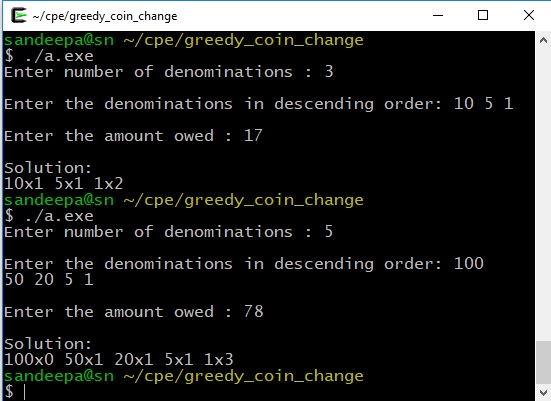# Greedy Change Making Program in C

### Problem Statement

Write a C program to solve ‘Change Making Problem’. Put simply, a solution to Change-Making problem aims to represent a value in fewest coins under a given coin system. This program was requested by one of readers on our Facebook Page.

#### Inputs to program

• Number of different denominations available
• List of numbers representing all the denominations
• Amount to represent in the given coin system

#### Output

The program should output a way to represent the given amount using fewest number of coins. For example. if you have coins of value 1, 2, 5 and 10 and want to represent 26, the program should output the correct solution 10×2 5×1 2×0 1×1

### Solution

The greedy approach is easy to understand and implement as well. We start with using the largest denomination coin/currency possible. Once the owed amount is less than the largest, we move to next largest coin, so on and so forth.

#### Assumptions

• Denominations are entered in descending order. That is largest one first. Example: 100, 50, 20, 5, 1. We can add a sort method if it’s not, a minor change.
• We always have denomination of 1(Just to make sure we have a solution for all the numbers).

#### Limitation

Greedy approach works best with Canonical Coin systems and may not produce optimal results in arbitrary coin systems. For example, if denominations are {4, 3, 1}, number 6 is represented as 4×1 3×0 1×2 by this program; taking 3 coins. The correct answer in this case is 4×0 3×2 1×0 with just 2 coins.

### The Program

This file contains bidirectional Unicode text that may be interpreted or compiled differently than what appears below. To review, open the file in an editor that reveals hidden Unicode characters. Learn more about bidirectional Unicode characters
 #include int main () { int num_denominations, coin_list, use_these, i, owed; printf("Enter number of denominations : "); scanf("%d", &num_denominations); printf("\nEnter the denominations in descending order: "); for(i=0; i< num_denominations; i++) { scanf("%d", &coin_list[i]); // use_these[i] = 0; } printf("\nEnter the amount owed : "); scanf("%d", &owed); for(i=0; i < num_denominations; i++) { use_these[i] = owed / coin_list[i]; owed %= coin_list[i]; } printf("\nSolution: \n"); for(i=0; i < num_denominations; i++) { printf("%dx%d ", coin_list[i], use_these[i]); } }

### Sample Output